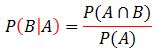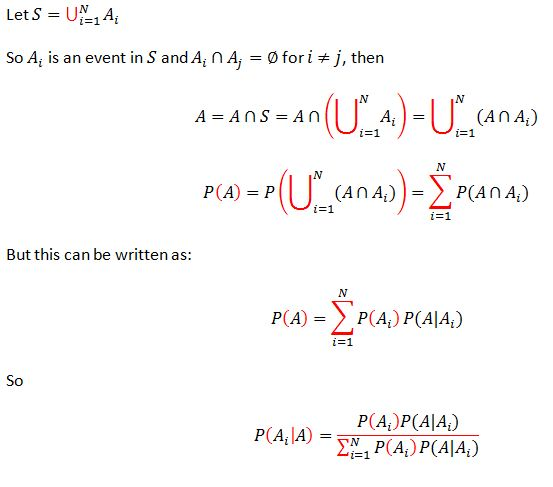### Introduction

In probability theory, Bayes’ theorem (also known as Bayes’ rule) is a useful tool for calculating conditional probabilities.In Bayes’ theorem, each probability has a conventional name:

• P(B│A) is the conditional probability of B, given A. It is also called the likehood.
• P(A) is the prior probability of A.
• P(B) is the prior probability of B.
• P(A│B) is the conditional probability of A, given B. It is also called the posterior probability because it is derived from or depends upon the specified value of B.

### To derive Bayes’ theorem

It is quite obvious to know the following two conditions:Then, P(A│B)P(B)=P(A∩B)=P(B│A)P(A), and finally, we get:### Extension

Extension 1:Note: P(B,C)= P(B∩C) is the probability of the interaction of B and C.

Extension 2:### For probability densities

There is also a version of Bayes' theorem for continuous distributions. It is somewhat harder to derive, since probability densities, strictly speaking, are not probabilities, so Bayes' theorem has to be established by a limit process; see Papoulis (citation below), Section 7.3 for an elementary derivation. Bayes' theorem for probability densities is formally similar to the theorem for probabilities:and there is an analogous statement of the law of total probability:As in the discrete case, the terms have standard names. f(x, y) is the joint distribution of X and Y, f(x|y) is the posterior distribution of X given Y=y, f(y|x) = L(x|y) is (as a function of x) the likelihood function of X given Y=y, and f(x) and f(y) are the marginal distributions of X and Y respectively, with f(x) being the prior distribution of X.

Here we have indulged in a conventional abuse of notation, using f for each one of these terms, although each one is really a different function; the functions are distinguished by the names of their arguments.

### References & Resources

• http://en.wikipedia.org/wiki/Bayes%27_theorem
• http://mathworld.wolfram.com/BayesTheorem.html
• Athanasios Papoulis (1984). Probability, Random Variables, and Stochastic Processes, second edition. New York: McGraw-Hill.